# Machine Learning - Confusion Matrix

If a classification system has been trained a confusion matrix will summarize the results (ie the error rate (false|true) (positive|negative) for a binary classification).

This is training error, there is may be overfitting.

The main diagonal indicates correct classification whereas everything off the main diagonal indicates a classification.

 Predicted class Actual Class True Positive False Negative False Positive True Negative

## Example

### Titanic Data Set

With a simple rule: If most females survived, then assume every female survives

Survived: Yes or No

 Predicted class Actual Class 233 81 109 468
• Total of good predictions : 233 + 468 = 701
• Total of bad predictions : 81 + 109 = 190
• Percentage of good prediction: 701 /( 701 + 190)*100 = 79%

## Documentation / Reference

• Bill Howe - Data Science Course

Recommended Pages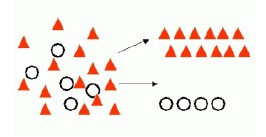Data Mining - (Classifier|Classification Function)

A classifier is a Supervised function (machine learning tool) where the learned (target) attribute is categorical (“nominal”) in order to classify. It is used after the learning process to classify...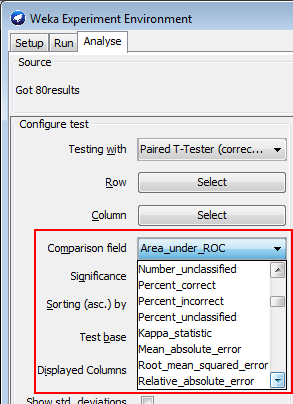Data Mining - (Parameters | Model) (Accuracy | Precision | Fit | Performance) Metrics

Accuracy is a evaluation metrics on how a model perform. rare event detection Hypothesis testing: t-statistic and p-value. The p value and t statistic measure how strong is the...R - Logistic Regression

logistic regression in R We have a call to GLM where we gives: the direction: the response, and the predictors, the family equals binomial. This parameter tells GLM to fit a logistic regression...Statistics - Bayes’ Theorem (Probability)

Bayesian probability is one of the different interpretations of the concept of probability and belongs to the category of evidential probabilities. In the Bayesian view, a probability is assigned to a...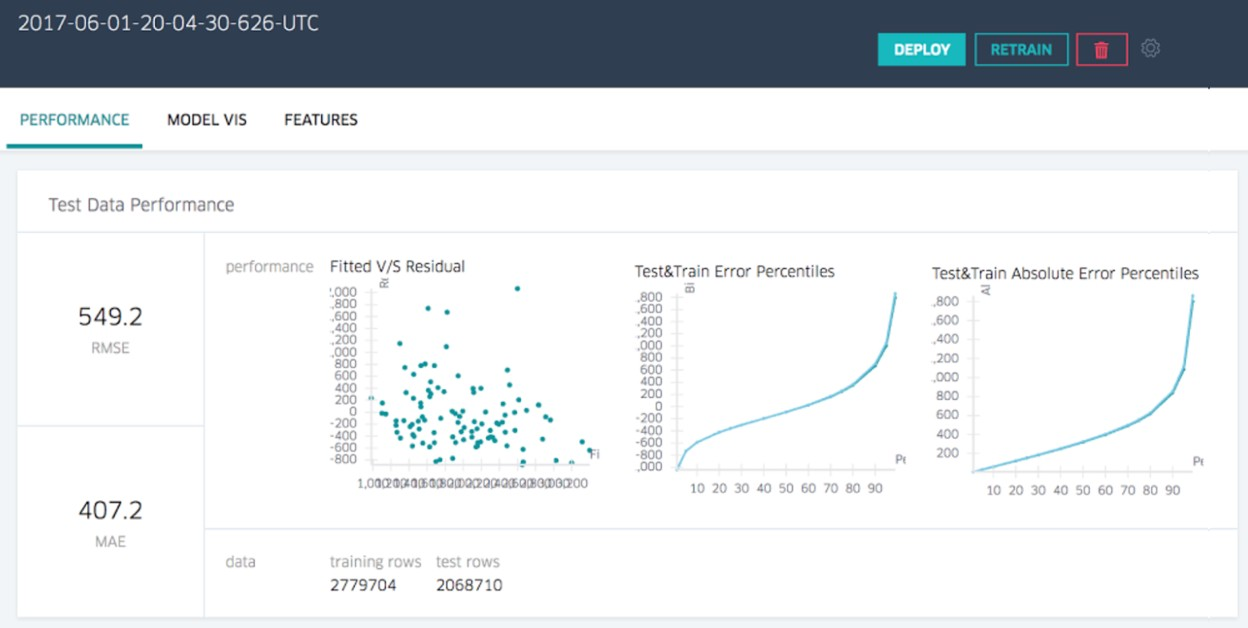Statistics - Model Evaluation (Estimation|Validation|Testing)

Evaluation is how to determine if the model is a good representation of the truth. Validation applies the model to test data in order to determine whether the model, built on a training set, is generalizable...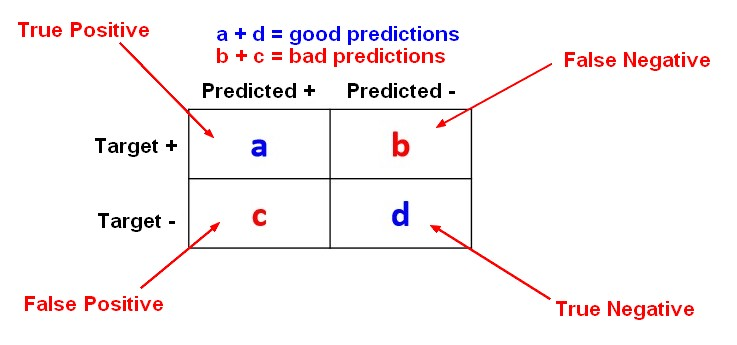Statistics Learning - (Error|misclassification) Rate - false (positives|negatives)

The error rate is a prediction error metrics for a binary classification problem. The error rate metrics for a two-class classification problem are calculated with the help of a Confusion Matrix. The...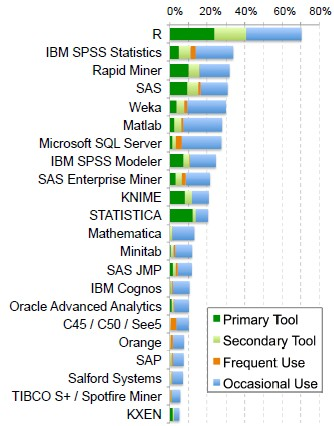Weka

is an open-source project in machine learning, Data Mining. is a comprehensive collection of machine-learning algorithms for data mining tasks written in Java....